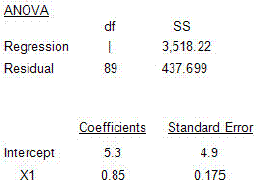### CFA Practice Question

There are 208 practice questions for this study session.

### CFA Practice Question

In comparing the relation of company sales with GDP, your regression software provides the following output:What must be the standard error of estimate over this period?
A. 2.22
B. 3.51
C. 4.91
Explanation: (SEE)2 = MSSE = SEE/(n - 2) = 437.699/89 = 4.91.

SEE = 2.22.